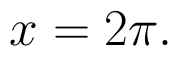Select Page

# Application of Integrals MCQ CBSE Maths 12 Science Solutions in English

Application of Integrals MCQ CBSE Maths 12 Science Solutions in English to enable students to get Solutions in a narrative video format for the specific question.

Expert Teacher provides Application of Integrals MCQ CBSE Maths 12 Science Solutions through Video Solutions in English language. This video solution will be useful for students to understand how to write an answer in exam in order to score more marks. This teacher uses a narrative style for a question from Application of Integrals not only to explain the proper method of answering question, but deriving right answer too.

Please find the question below and view the Solution in a narrative video format.

Question:

Solution Video in English:

## Similar Questions from CBSE, 12th Science, Maths, Application of Integrals

Question 1 : Find the area bounded by the curve y = sin x between x = 0 and(View Answer Video)

Question 2 : Using integration find the area of region bounded by the triangle whose vertices are (-1, 0), (1, 3) and (3, 2). (View Answer Video)

Question 3 : Using integration, find the area bounded by the tangent to the curveat the point (2, 1) and the lines whose equations are x = 2y and x = 3y - 3. (View Answer Video)

Question 4 : Find the area of the region bounded by the curveand. (View Answer Video)

Question 5 : Find the area enclosed by the parabolaand the line 2y = 3x + 12.   (View Answer Video)

### Probability

Question 1 : If E and F are two events such that,, Find P(E or F).         (View Answer Video)

Question 2 :  A and B throw a pair of dice alternatively, till one of them gets a total of 10 and wins the game. Find their respective probabilities of winning, if A starts first.   (View Answer Video)

Question 3 : If E and F be two evets such that, findif E and F are independent events. (View Answer Video)

Question 4 :  A coin is tossed three times. Find P(F/E), where E : at most two tails and F : atleast one tail.  (View Answer Video)

Question 5 : An urn contains 4 balls. Two balls are drawn at random from the urn (without replacement) and are found to be white. What is the probability that all the four balls in the urn are white?  (View Answer Video)

### Differential Equations

Question 1 : Solve the differential equation(View Answer Video)

Question 2 : Write the differential equation formed from the equation y = mx + c, where m and c are arbitrary constants.

Question 3 : Determine degree of(View Answer Video)

Question 4 : Obtain the differential equation of all the circles of radius r.   (View Answer Video)

Question 5 : Find the solution of the differential equation(View Answer Video)

### Vector Algebra

Question 1 : L and M are two points with position vectorsandrespectively. Write the position vectors of a point N which divides the line segment LM in the ratio 2:1 externally.   (View Answer Video)

Question 2 :  Find the position vector of c which divides the line segment joining A & B whose position vectors areandinternally in the ratio
Question 3 : Find the sum of the vectorsand(View Answer Video)
Question 4 : Find the area of the parallelogram whose adjacent sides are determined by the vectorsand(View Answer Video)
Question 5 : Write the position vector of the point which divides the join of points with position vectorsandin the ratio 2:1.  (View Answer Video)